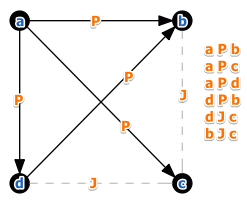# ML Wiki

## Partial Order

This is a preference structure for Modeling Preferences in MCDA that includes $J$ - the Incomparability relation.

Assume:

• there are different experts $\{1, 2, 3\}$
• they evaluate 4 projects $a, b, c, d$
• investment $a$ is preferred to investment $b$ if estimates from $a$ are higher than from $b$ (or $a$ dominates $b$)
• i.e. there is Unanimity between the experts
$a$ $b$ $c$ $d$
1 10 8 7 6
2 9 7 5 6
3 12 8 9 4

We can infer the following relations:

• $a \ P \ b$ because all three experts agree
• but $b \ J \ c$:
• 1st expert say $b \ P \ c$
• but 3rd say $c \ P \ b$
• therefore we cannot compare $a$ and $b$so we have partial order:

• $P$ is transitive
• and $J$ is not empty